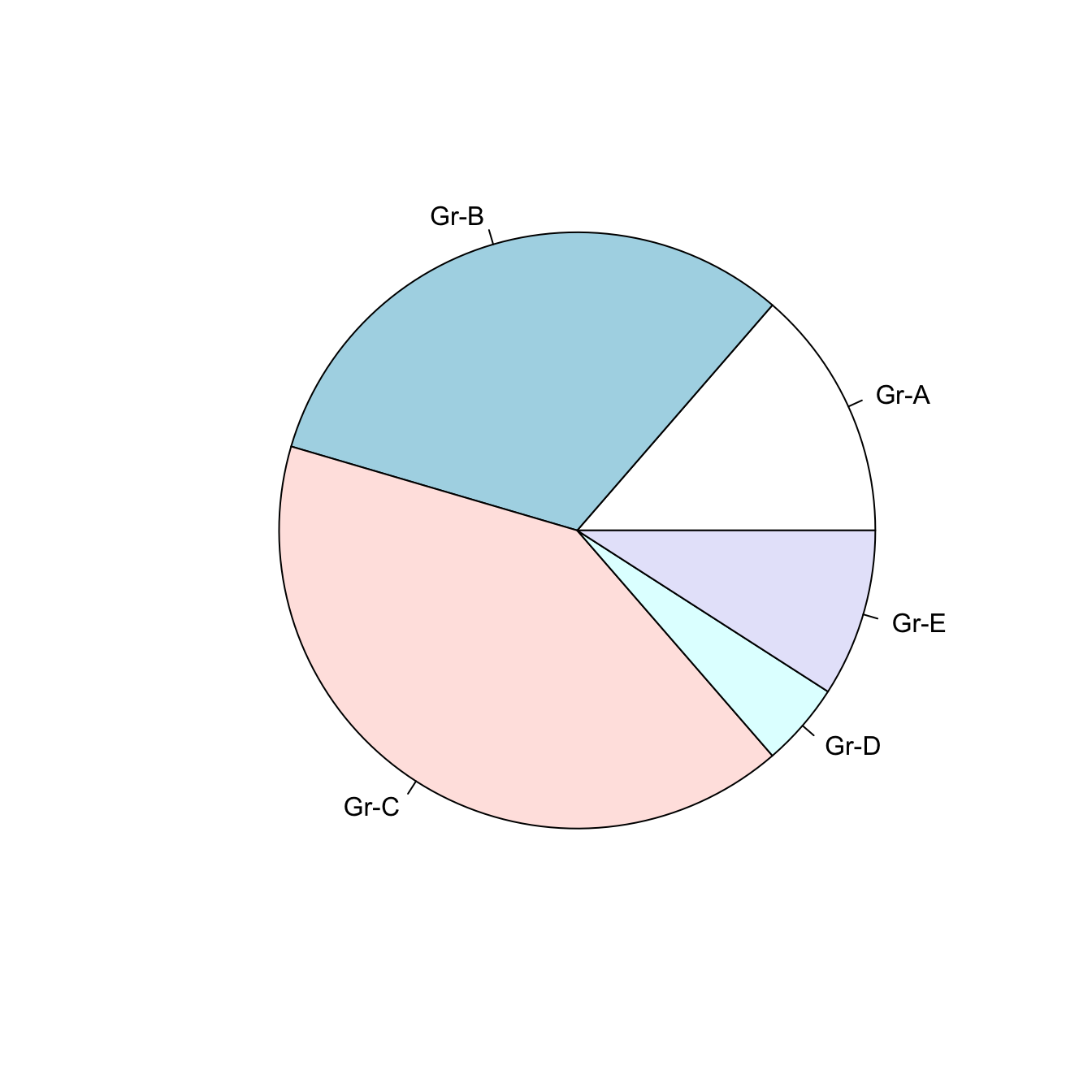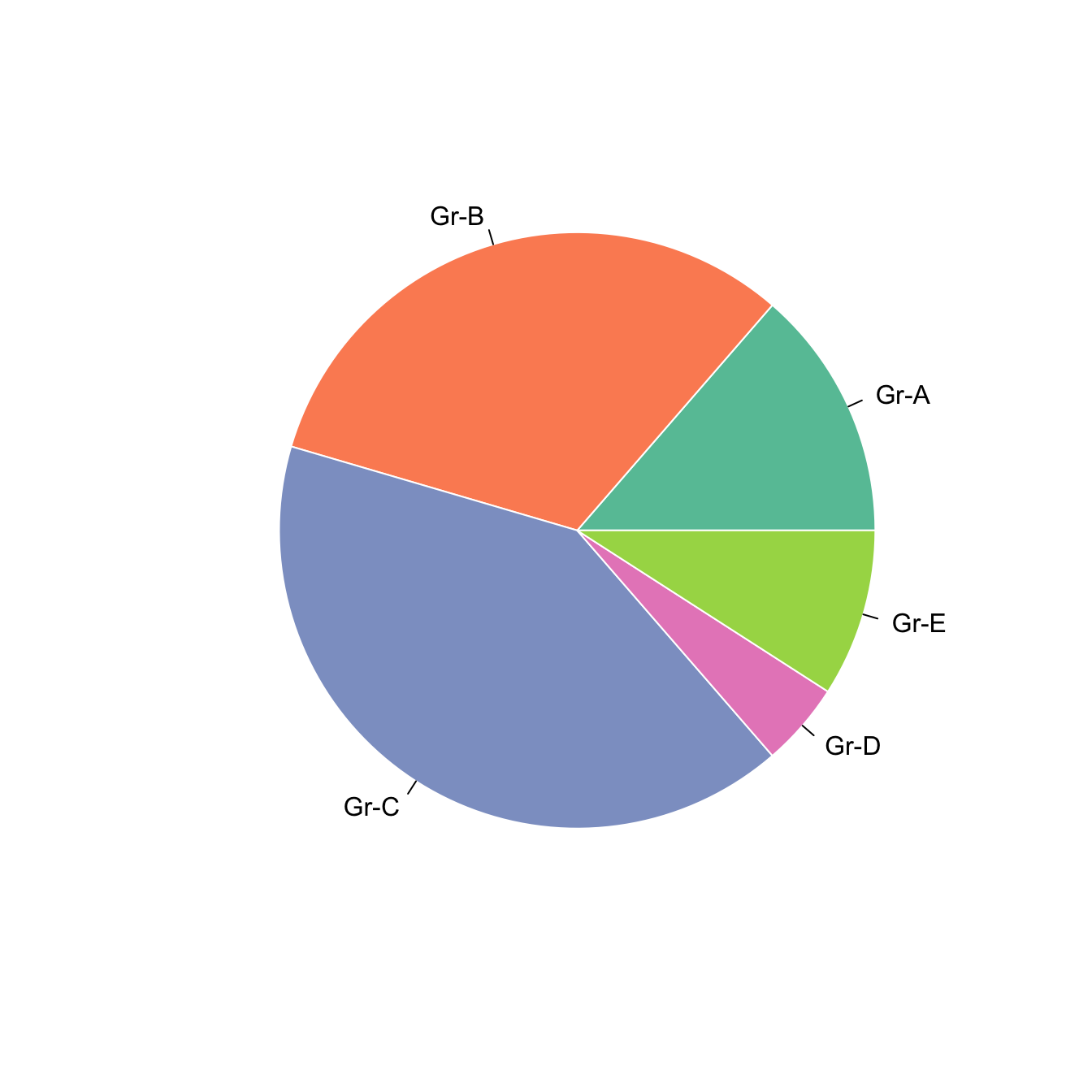# Pie plot with base R

A pie chart is a circle divided into sectors that each represent a proportion of the whole. This page explains how to build one with basic `R` commands.

# Most basic pie chart with `pie()`

`R` natively offers the `pie()` function that builds pie charts. The input is just a numeric variable, each value providing the value of a group of the piechart.

Important note: pie chart are widely known as a bad way to visualize information. Check this post for reasons and alternatives.``````# Create Data
Prop <- c(3,7,9,1,2)

# Make the default Pie Plot
pie(Prop)``````

# Change labels with `labels`

Provide a vector of labels to the `labels` argument to add names to piechart groups:``````# You can also custom the labels:
pie(Prop , labels = c("Gr-A","Gr-B","Gr-C","Gr-D","Gr-E"))``````

# Non-circular piechart with `edges`

Decrease the value of the `edges` argument to get angles around your piechart.``````# If you give a low value to the "edge" argument, you go from something circular to a shape with edges
pie(Prop , labels = c("Gr-A","Gr-B","Gr-C","Gr-D","Gr-E") , edges=10)``````

# Add stripes with `density`

The `density` arguments adds stripes.

You can control the angle of those stripes with `angle`.``````# The density arguments adds stripes. You can control the angle of this lines with "angle"
pie(Prop , labels = c("Gr-A","Gr-B","Gr-C","Gr-D","Gr-E") , density=10 , angle=c(20,90,30,10,0))``````

# Color with `col` and `border`

Change group color with `col`, and border color with `border`.

Here, the `RcolorBrewer` package is used to build a nice color palette.``````# Prepare a color palette. Here with R color brewer:
library(RColorBrewer)
myPalette <- brewer.pal(5, "Set2")

# You can change the border of each area with the classical parameters:
pie(Prop , labels = c("Gr-A","Gr-B","Gr-C","Gr-D","Gr-E"), border="white", col=myPalette )``````

Related chart types

## Contact

This document is a work by Yan Holtz. Any feedback is highly encouraged. You can fill an issue on Github, drop me a message on Twitter, or send an email pasting yan.holtz.data with gmail.com.